# Quiescent voltage of op-amps

• Engineering
• peasngravy
• Featured
You don't calculate Vin--it's a given. You assume a sine wave of voltage at a frequency of 1 Hz is applied to Vin. I like to assume that the voltage of the input is 1 volt AC, but any voltage is OK. Then you calculate the way this voltage signal is amplified as it passes through the circuit. The voltage at Vout is your final result. The voltage gain is then given by Av = Vout/Vin.

In the real world the voltage at Vin due to the sensors will probably be on the order of millivolts, but for your problem since it's just math, you can assume any Vin. Since the circuit is being treated as if it's perfectly linear with unlimited output voltage capability from the opamps for the purpose of doing the math, any Vin will do.

Concerning C4 and C6, it is NEVER a good idea to hang a capacitor directly on the output of an op-amp. I will defend your original statement about them being put there by an idiot.
-
Now, that being said, many years ago I was likely guilty of doing that exact same thing in some homebrew project before I knew better. If something like this were to ever help prevent an oscillation it is likely an example of 2 wrongs making a right. And that in and of itself is still wrong.
Yes, however, in this case it doesn't matter for stability. That pole is around 1MHz and these circuits cross-over at 1-2KHz. Still, I have no idea what they think it's supposed to do.

Yes, however, in this case it doesn't matter for stability. That pole is around 1MHz and these circuits cross-over at 1-2KHz. Still, I have no idea what they think it's supposed to do.

Based on the frequency response of the circuit, it appears that the operating frequency is about 1-2 Hz, not 1-2 kHz.

You don't calculate Vin--it's a given. You assume a sine wave of voltage at a frequency of 1 Hz is applied to Vin. I like to assume that the voltage of the input is 1 volt AC, but any voltage is OK. Then you calculate the way this voltage signal is amplified as it passes through the circuit. The voltage at Vout is your final result. The voltage gain is then given by Av = Vout/Vin.

In the real world the voltage at Vin due to the sensors will probably be on the order of millivolts, but for your problem since it's just math, you can assume any Vin. Since the circuit is being treated as if it's perfectly linear with unlimited output voltage capability from the opamps for the purpose of doing the math, any Vin will do.

Well I wish I asked you that much sooner as I spent a long time on google trying to work it out :D

In pspice you simply connect an AC voltage source to Vin. Set the parameters of the source for your desired frequency and voltage amplitude.

Given that it's an ideal op-amp and the gain can't be anything other than unity, then all inputs and outputs of the op-amps are 2vdc?

And I just need to perform a small signal ac analysis to work out the overall voltage gain?

•berkeman
Given that it's an ideal op-amp and the gain can't be anything other than unity, then all inputs and outputs of the op-amps are 2vdc?

And I just need to perform a small signal ac analysis to work out the overall voltage gain?

Yes and Yes.

•peasngravy and berkeman
Have you tried to derive the non-inverting gain of just the first opamp stage using equivalent impedance of the C3 R5 pair as Rf, and the C2 R4 pair equivalent as Rin? Ignore the effect of C9, R2, R1 and R12; they are just a voltage divider in front of the "+" input of the first opamp.

Have you tried to derive the non-inverting gain of just the first opamp stage using equivalent impedance of the C3 R5 pair as Rf, and the C2 R4 pair equivalent as Rin? Ignore the effect of C9, R2, R1 and R12; they are just a voltage divider in front of the "+" input of the first opamp.

I did calculate those pairs - using 1hz frequency, I got Z values of 16.06Meg and 12.34K for those which gave me a gain of 1301 (1+(rf/rin))

I checked this on circuitlab (with an AC source at 1V and 1 hz) and found the value did not match up so I am a bit lost now. This gave me a gain of around 135. These are the values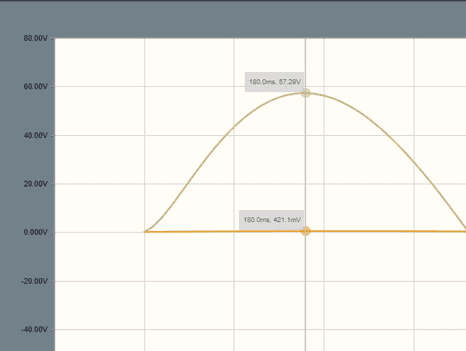I did calculate those pairs - using 1hz frequency, I got Z values of 16.06Meg and 12.34K for those which gave me a gain of 1301 (1+(rf/rin))

If you'll show your work, I can see where you went wrong and suggest a fix.

Sure - so using 1hz as the frequency, ω = 2πf = 6.283
C2 = 22uF
R4 =10k
|Z| = √ R² +(1/(ωC²))
|Z| = √ 10k² +(1/(6.283*0.000022²))
|Z| = 12342 for Rin

I just noticed where I went wrong actually, and I used this equation for both pairs, but one is in series and the other is parallel.

So the other pair using this formula
|Z|=1√(1R)2+(ωC)2

Gives me 2.179Meg for Rf

Av = 1+(Rf/Rin)
= 1+(2179000/12342)
=176

Which is a bit closer but still slightly out

You need to carry more digits in your arithmetic.

When I carry out this division: (2179000/12342) I get 176.55161238
After adding 1, I get 177.55161238

But carrying more digits in all the calculations, I get the desired quotient as: 2179278.18/12342.4196 = 176.568148
The after adding 1 I get: 177.568148

What do you think the correct result is, and where did you get the value you believe is correct?

You will get a tiny error in your calculations because you aren't using complex arithmetic. When you add 1 to the quotient of the two impedances, the impedances should be kept in complex form for the exactly correct final result which is: 177.4516107

I don't think spice will give you that many digits, but that is the exact correct result.

Your problem statement asks you for an estimate. I would say you have a very good estimate, but do carry a few more digits so your result is 177.55, not 176.

Next, what is the ratio of the voltage divider made of C9, R3, R1 and R12.

Then, show your work for the second opamp stage.

•peasngravy
You need to carry more digits in your arithmetic.

When I carry out this division: (2179000/12342) I get 176.55161238
After adding 1, I get 177.55161238

But carrying more digits in all the calculations, I get the desired quotient as: 2179278.18/12342.4196 = 176.568148
The after adding 1 I get: 177.568148

What do you think the correct result is, and where did you get the value you believe is correct?

You will get a tiny error in your calculations because you aren't using complex arithmetic. When you add 1 to the quotient of the two impedances, the impedances should be kept in complex form for the exactly correct final result which is: 177.4516107

I don't think spice will give you that many digits, but that is the exact correct result.

Your problem statement asks you for an estimate. I would say you have a very good estimate, but do carry a few more digits so your result is 177.55, not 176.

Next, what is the ratio of the voltage divider made of C9, R3, R1 and R12.

Then, show your work for the second opamp stage.

I think the number around 177 is mathematically correct so it has to be the correct value - the pspice was simply to use a as a bit of a guide and I was hoping they'd be a bit closer, that's all.

For the voltage divider you mentioned, can I use the Z value of C9 and R3 in there?

The signal coming in at Vin passes through the series combination of C9 and R3. Calculate the |Z| of those two and that forms a voltage divider followed by a resistance to ground equal to the parallel combination of R1 and R12, with the output of the divider applied to the "+" input of the opamp. You will multiply the voltage divider ratio (which will be less than unity) times the gain of the first opamp. That will give the gain from Vin to the first opamp output.

Then you will calculate the gain of the second opamp and multiply that factor into get the overall gain.

The signal coming in at Vin passes through the series combination of C9 and R3. Calculate the |Z| of those two and that forms a voltage divider followed by a resistance to ground equal to the parallel combination of R1 and R12, with the output of the divider applied to the "+" input of the opamp. You will multiply the voltage divider ratio (which will be less than unity) times the gain of the first opamp. That will give the gain from Vin to the first opamp output.

Then you will calculate the gain of the second opamp and multiply that factor into get the overall gain.

So R1 and R12 have a ratio of 0.4 - I am not quite sure how to factor in the impedance of c9 and r3.

The ratio of 0.4v is quite close to what my pspice model suggests as the input (430mv)

After that - I think I have to calculate the series capacitance of c5+c8 and then use that figure to calculate the impedance of the rc series along with r6 which should be my Rin for the second op amp?

So R1 and R12 have a ratio of 0.4 - I am not quite sure how to factor in the impedance of c9 and r3.

The ratio of 0.4v is quite close to what my pspice model suggests as the input (430mv)

After that - I think I have to calculate the series capacitance of c5+c8 and then use that figure to calculate the impedance of the rc series along with r6 which should be my Rin for the second op amp?
The key problem for solving the 2nd amp is dealing with the C5/C8 network at the input. Because the virtual ground of the 2nd amp will keep the AC (signal) voltage = 0 at the input (BTW, don't confuse this with the non-zero DC voltage there), this problem amounts to given a signal voltage applied at the 1st amp output, what will the current flow be into the 2nd amp. That current will have to flow through the feedback components to create the output voltage. Something like this picture: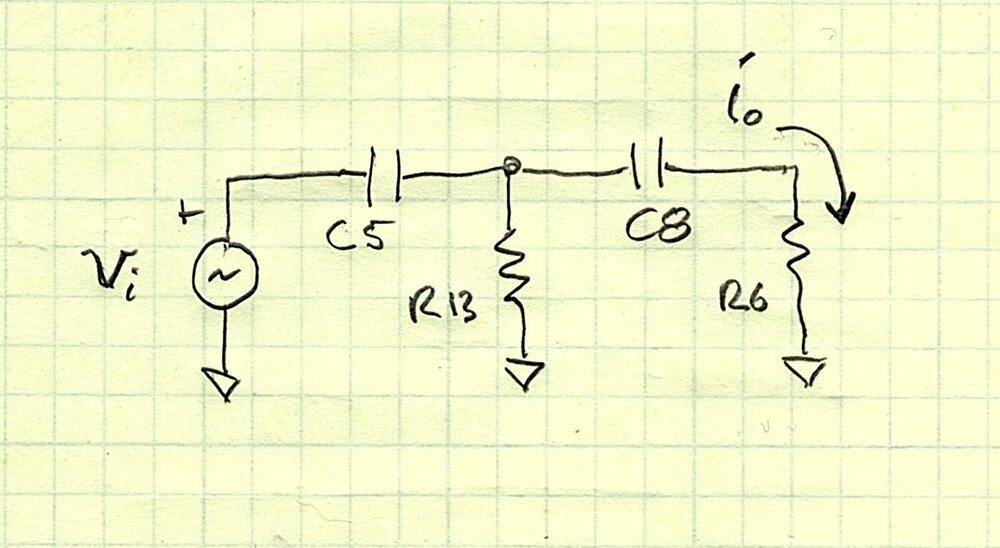edit: note that you will end up with an equivalent impedance Zeq = Vi(jω)/Io(jω), although I phrased it as an admittance (i.e. find Io given Vi); whatever, there sort of the same, in a reciprocal fashion, LOL. Then you can pretend it is a two terminal complex impedance, if you like to think of things that way. BTW this Zeq simplification only works for Vi and Io, it won't be correct if you want to know other things in the network, like the current through R13, etc.

Last edited:
•peasngravy
So R1 and R12 have a ratio of 0.4 - I am not quite sure how to factor in the impedance of c9 and r3.

The ratio of 0.4v is quite close to what my pspice model suggests as the input (430mv)

After that - I think I have to calculate the series capacitance of c5+c8 and then use that figure to calculate the impedance of the rc series along with r6 which should be my Rin for the second op amp?

The ratio of R1 and R12 is not relevant. Forget about the quiescient DC voltages in the circuit. Have a look here: https://en.wikipedia.org/wiki/Voltage_divider

In Figure 1 on that page let Z1 be the impedance magnitude of the series combination of C9 and R3, and let Z2 have the value of R1 and R12 in parallel. Then if 1 volt is applied to Vin in Figure 1, the output voltage will be applied to the "+" input of the first opamp. Multiply that value times the gain of the opamp and you have the gain from Vin of your circuit to the output of the first opamp. The voltage at the output of the first opamp is applied to the left side of C5. Then you calculate the gain from that point to the output of the second opamp.

To calculate the gain of the second opamp, follow the advice of DaveE and calculate the current in R6. Multiply that current times the impedance magnitude of the C7 R9 pair (remember that they're in parallel) and you have the voltage Vout at the output of the second opamp.

•peasngravy
The key problem for solving the 2nd amp is dealing with the C5/C8 network at the input. Because the virtual ground of the 2nd amp will keep the AC (signal) voltage = 0 at the input (BTW, don't confuse this with the non-zero DC voltage there), this problem amounts to given a signal voltage applied at the 1st amp output, what will the current flow be into the 2nd amp. That current will have to flow through the feedback components to create the output voltage. Something like this picture: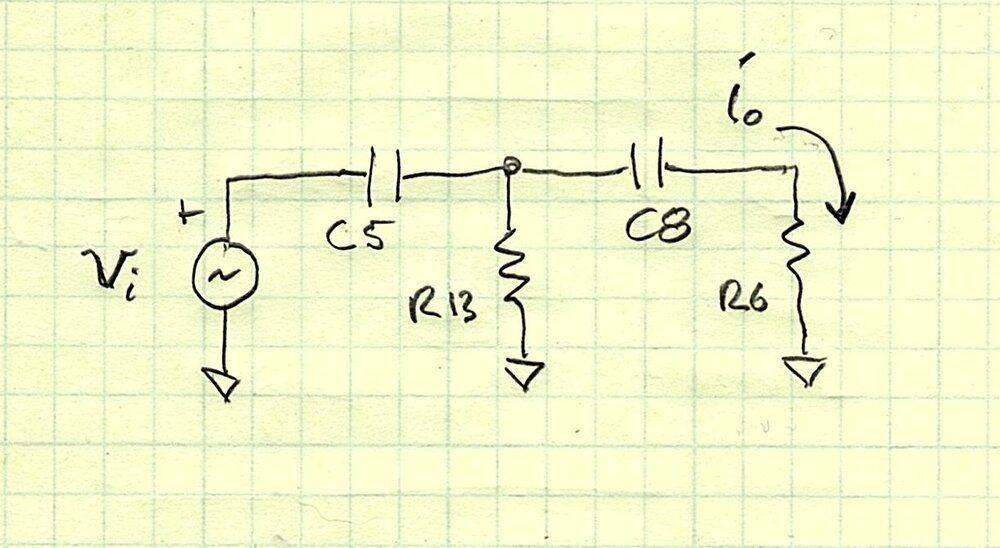edit: note that you will end up with an equivalent impedance Zeq = Vi(jω)/Io(jω), although I phrased it as an admittance (i.e. find Io given Vi); whatever, there sort of the same, in a reciprocal fashion, LOL. Then you can pretend it is a two terminal complex impedance, if you like to think of things that way. BTW this Zeq simplification only works for Vi and Io, it won't be correct if you want to know other things in the network, like the current through R13, etc.

As this is time dependent due to the capacitors, is there any particular point in time I should choose for this? Or does jω negate the need for this?
I can't find a way to use j, excel and my calculator do not like it

Edit - i will show my working up to this point. I am very close now, thank you allfor guiding me this far

Series combination of C9 and R3 forms ad RC series with the magnitude of Z, which forms a voltage divider with the parallel combination of R1 and R12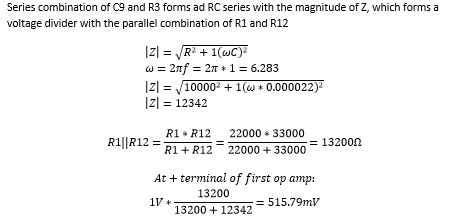So AC voltage at output of first op amp = 515.79 * 177.658 = 91.765V

I had written this in word and for some reason the equations don't copy over so had to screenshot

#### Attachments

Last edited:
Your procedure is correct, but there are some small arithmetic errors.

The value 515.79 should be .51579 in the following calculation, but even then the calculation you show is giving a slightly wrong result: .51579 * 177.658 = 91.765V should be as shown below: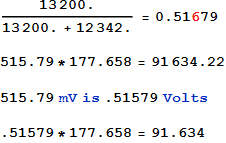But using .51679 instead of .51579 you should get:

.51679 * 177.658 = 91.812

Next calculate the C5 R13 C8 R6 impedance input to the second opamp. DaveE gave the procedure.

Last edited:
I can't find a way to use j, excel and my calculator do not like it
What calculator are you using? My HP-42 (simulator on my smartphone) has "Complex" mode.

And Excel has a COMPLEX function. Just look it up in Excel Help: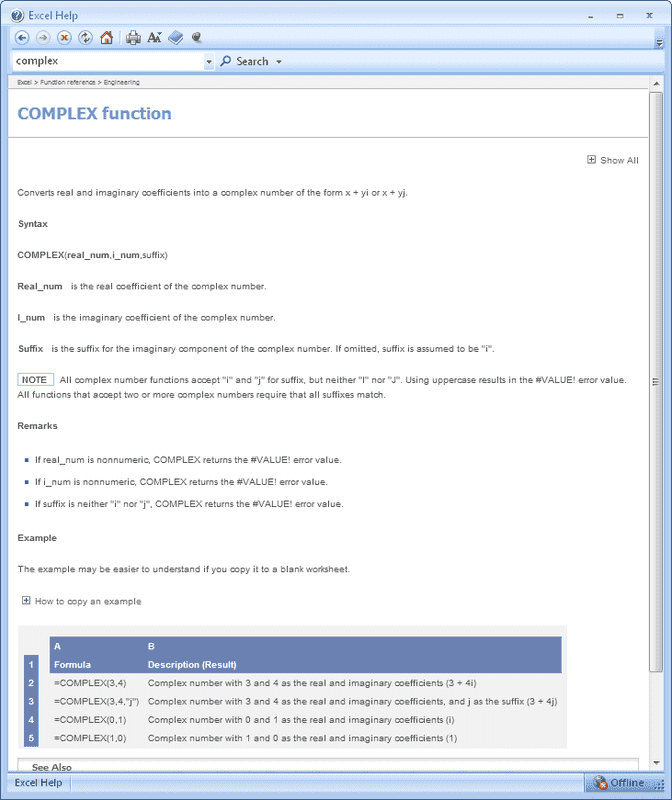I am very close now, thank you allfor guiding me this far•peasngravy
Are C5/R13 and C8/R6 just two impedances in parallel?

Are C5/R13 and C8/R6 just two impedances in parallel?
Calculate an equivalent for C8 R6 and then that equivalent is in parallel with R13 giving another equivalent. Then that equivalent forms a voltage divider with C5. The output of that voltage divider is a voltage which drives a current through C8 and R6. Divide the voltage out of the voltage divider by the equivalent impedance magnitude of C8 R6, giving you a current. Multiply that current times the equivalent impedance magnitude of C7 R9 and you have the gain of the second opamp.

•peasngravy
C8 R6 = 33783.64566
C8 R6 in parallel with R13 = (33783.64566*10000)/(33783.64566+10000) = 7716.042178

Using this formula: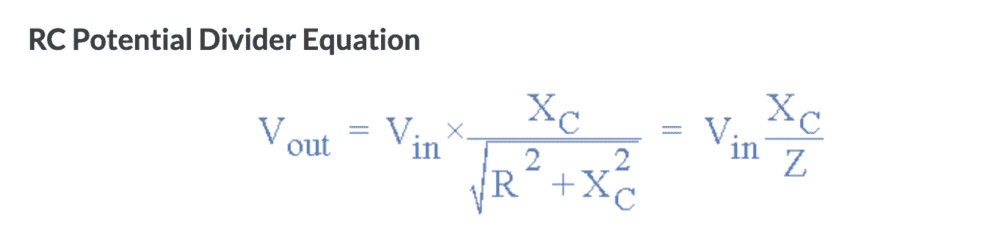With Xc of C5 being 1/(2 pi fC) = 3386.2754

Vout = 91.812 * (3386.2754/7716.042178) = 0.4388617V

Dividing by Z of C8 R6, for current = 0.00001299A
Impedance of C7 R9 = 990580.99 ohm

Gain of 2nd op amp = 0.00001299 * 990580.99 = 12.86800311

Gain of 2nd op amp * Vin = 1170V

Last edited:
Thanks for that - i was trying to simply use a cell to calculate the square root of -1 which obviously is not possible, but I thought excel would recognise it, I didn't realize there was a specific function

C8 R6 = 33783.64566
C8 R6 in parallel with R13 = (33783.64566*10000)/(33783.64566+10000) = 7716.042178

Using this formula:
View attachment 273118
You are correct up to this point, but this formula is not what we want to use here.

With Xc of C5 being 1/(2 pi fC) = 3386.2754

Vout = 91.812 * (3386.2754/7716.042178) = 0.4388617V
I'll show corrections in red.

Use the voltage divider formula from Wikipedia with the appropriate impedance magnitudes:
Vout = 1 volt *(7716.042178)/ (3386.2754+7716.042178) = 1 volt * .6949968 = .6949968
We will multiply by 91.812 later.

Dividing by Z of C8 R6, for current = .6949968/33783.64566 = .00002057198
Impedance of C7 R9 = 990580.99 ohm

Gain of 2nd op amp = 0.00002057198 * 990580.99 = 20.3782

Overall circuit gain = Gain of 2nd op amp * Vin = 20.3782 * 91.812 = 1870.96
The Vin used just above isn't the Vin of the overall circuit; it's the output of the first opamp.

This is somewhat in error because impedance magnitudes were used in calculations rather than
the complex impedances. This was done because apparently your course work hasn't taught you
how to use complex arithmetic when capacitors are in the circuit, but using impedance magnitudes is better than doing nothing. I suppose the final result can be called a reasonable estimate given the use of impedance magnitudes.

If you're interested, I will show how I solved the complete network using nodal analysis.

Last edited:
Thank you!

Yes I'd be interested to see that if you have the time. I find this method of learning much better than simply reading pages and pages of notes

Thank you!

Yes I'd be interested to see that if you have the time. I find this method of learning much better than simply reading pages and pages of notes
Here is how I solved your problem circuit; I used the nodes shown: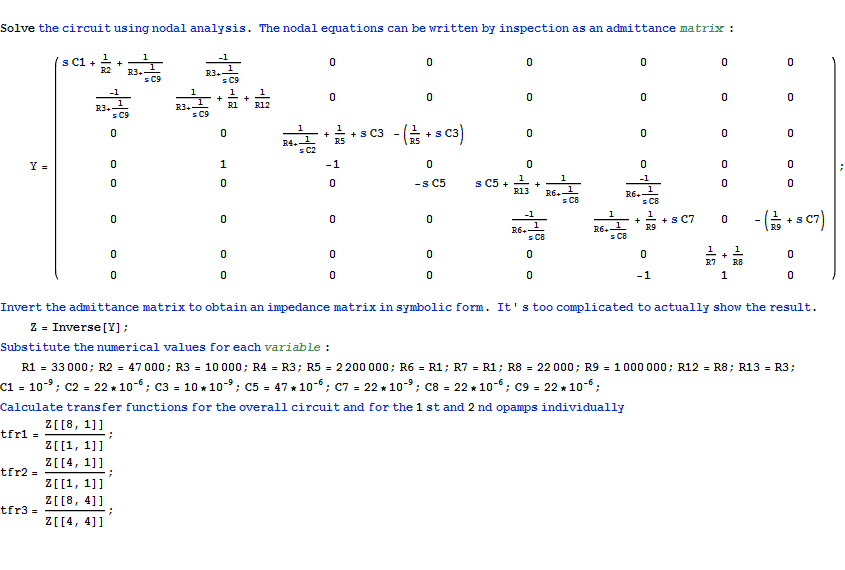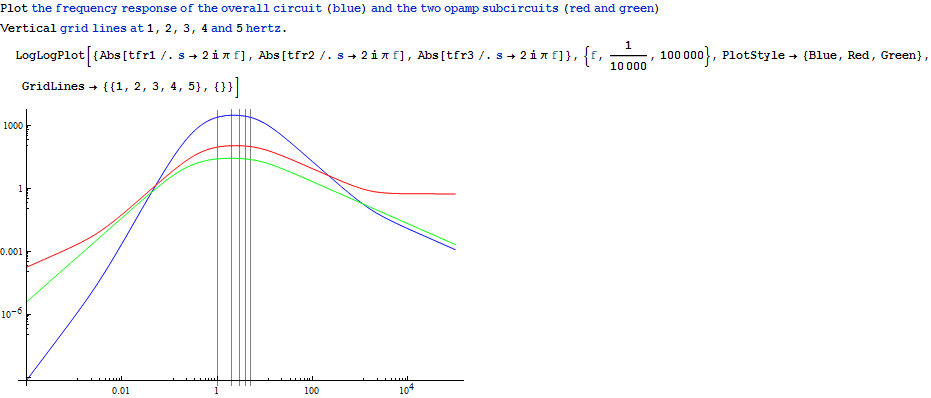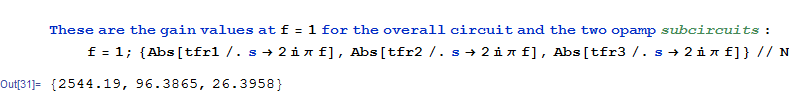The amount of complex algebra involved in this procedure would be impossible to do without
the help of a modern computer running a powerful mathematical software.Last edited:
That is very impressive - what software is that? At first glance it looks pretty complex but I think i could have fun messing around with that

That is very impressive - what software is that? At first glance it looks pretty complex but I think i could have fun messing around with that
It's Mathematica. There are other programs with similar capability such as Matlab, Mathcad, Maple; these are commercial programs costing real money. There are free programs such as Macsyma, Octave and others.

Are C5/R13 and C8/R6 just two impedances in parallel?
No, not really. First, because of the virtual ground of the op-amp, R6 & C8 can be treated as series impedances (as shown in my previous sketch).

The analysis of the rest of this network is more complicated. Frankly, at your level, it's really hard. So, before you can finish the problem you really have to deal with just the solution to this network; which we would call a "T-section", BTW. All of the other impedances you have calculate so far have terms on the order of ω, like (1 + jω⋅R⋅C). This T-section, when solved exactly, will have a quadratic term, with order ω2; something that looks like (1 + jω/(Q⋅ωo) + (jω/ωo)2). Frankly, this is a PIA to deal with. Sometimes if the circuit values are really different in their frequency response, you can approximate this with two 1st order functions. like (1 + jω⋅τ1)⋅(1 + jω⋅τ2). Unfortunately, I don't think you can do that for the component values you were given. Plus there's a whole additional lesson(s) involved in learning these techniques.

So, how should we proceed? Honestly, I don't think your instructor intends for you to solve this exactly. Or if he thinks you should, then he doesn't really understand the complexity of an exact solution. I'm a bit confused by the original question, myself. You said in post #1 "find the frequency of operation", I don't really know what he means by this. The frequency of operation implies some range of frequencies that the circuit is exposed to, or that the designer cared about. I would guess that he is asking for the what I would call the center frequency of the filter response. But, then I don't know why he didn't say that.

Anyway, I think as a result, we have assumed that you need an exact solution, it's the only way we can be sure to be correct. Maybe that's not what you need?

It's possible that he intended for you to refer to some reference online or in lectures about the solution to this "2nd order T-section high-pass filter", which, practically always are over simplified. Or maybe he expects you to give a rough approximation or do a simulation. None of these will really teach you how to really design or solve these filters the way professionals do ought to.

So, while I don't normally like to give an answer, as opposed to helping people figure problems out themselves, In this case I'll share my notes (not a compete solution), just so you can see the complexity and the general form of the answer to the hardest part of this circuit. Note that I didn't intend for this to be educational, it's just what I did for myself. The key step for me is using Thevenin's theorem to create the second schematic, which has reduced complexity, as well a s the current divider rule. These may seem cryptic to you if you haven't studied them yet. Also, don't be confused by my use of s (s = j⋅ω), a trivial substitution. Finally, I didn't check it twice, so I could have made a mistake, although it looks right vis-à-vis units and intuition.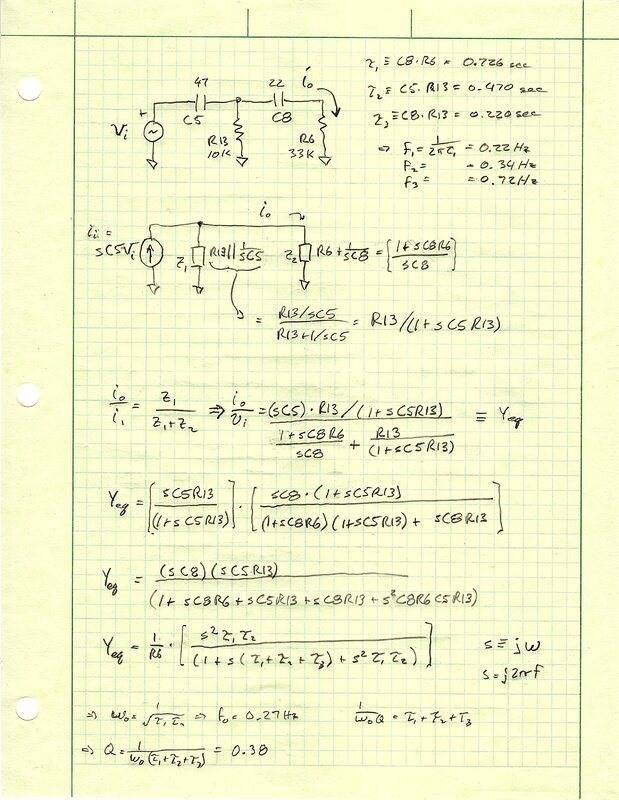Last edited:
•berkeman
And the rest of my notes below. Sorry, I know y'all can't read this, but that's how lazy engineers work in real time, LOL. Plus we all speak the same language but with different dialects.

There are a couple of things to point out though. The big one is that the problem really needs to be broken down into separate sections, otherwise the analysis is too hard and you won't understand the results anyway. Sort of like putting it into a simulator and getting answers without much understanding of why. The other point is that the algebra is simplified if you know the form you want it to end up in, which IMO, should ALWAYS be a "factored pole-zero" form, also sometimes called the canonical form. This allows you to maintain the functional description of each subsection and is trivial to combine into the whole transfer function by multiplication (which I didn't do).

Still not finished, I'm loosing interest. Theoretically there are two quadratic responses, but the one in the first amp has such low Q, it can be simplified to two real poles (which I also didn't do). Plus I totally ignored the inversion at the last amp, which is why you ALWAYS have to check for dumb mistakes, we all make them, nearly every time.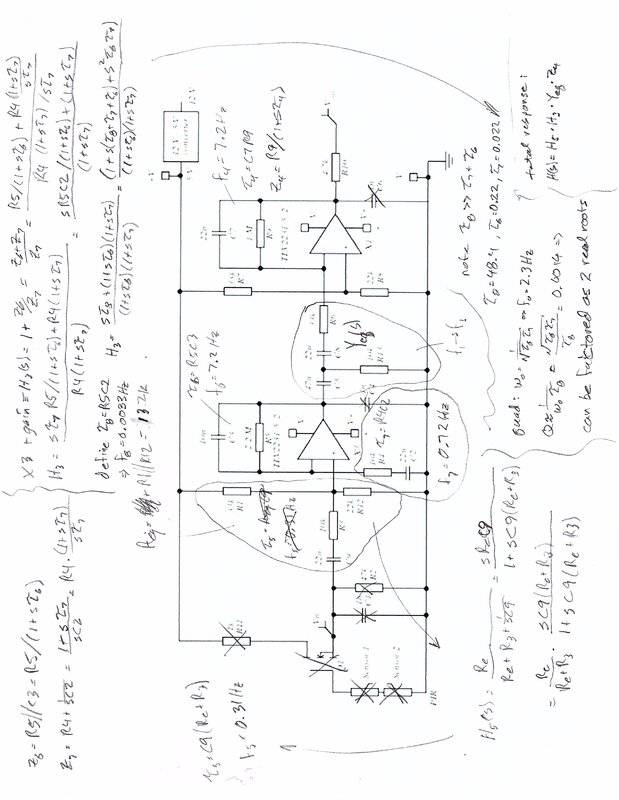Last edited:
And the rest of my notes below. Sorry, I know y'all can't read this, but that's how lazy engineers work in real time, LOL. Plus we all speak the same language but with different dialects.

There are a couple of things to point out though. The big one is that the problem really needs to be broken down into separate sections, otherwise the analysis is too hard and you won't understand the results anyway. Sort of like putting it into a simulator and getting answers without much understanding of why. The other point is that the algebra is simplified if you know the form you want it to end up in, which IMO, should ALWAYS be a "factored pole-zero" form, also sometimes called the canonical form. This allows you to maintain the functional description of each subsection and is trivial to combine into the whole transfer function by multiplication (which I didn't do).

Still not finished, I'm loosing interest. Theoretically there are two quadratic responses, but the one in the first amp has such low Q, it can be simplified to two real poles (which I also didn't do). Plus I totally ignored the inversion at the last amp, which is why you ALWAYS have to check for dumb mistakes, we all make them, nearly every time.

View attachment 273138

Have you read posts #57 through #62? The original problem only called for estimates of gain.

Have you read posts #57 through #62? The original problem only called for estimates of gain.
Sort of, enough to get the big picture, but not in detail. I know how these stories go. Nothing wrong with nodal analysis. If you are really familiar with it you can see some of the natural portioning in the matrix form (diagonals, and such). You get an A+ in my book because your matrix is full of variables (R3, C5, et. al.). It's a great way to get the answer, that's why all of the simulators are based on it. However, it isn't great for me at understanding the relationship between response parameters and the individual components.

I prefer the "design oriented" analysis approach championed by my prof. R.D. Middlebrook. Don't just get the answer, understand what each part does. But as I said, we do often speak different dialects. Your result is a Mathematica plot, mine is a formula that you could plot with a pencil and paper (if you are familiar with the canonical forms), or with any piece of math SW.

But, gain is a function of frequency in these circuits.

I guess the part of this thread that I didn't really follow was why (somewhere in posts #50 - 60) we decided that the answer will be found by evaluating the gain at 1 Hz. Probably I missed the justification, or perhaps a computer told you that 1 Hz was the "frequency of operation".

The way I look at these problems, you can't really identify that frequency until you understand how all of the various poles and zeros in the response interact. OK, actually, I still don't know what that phrase means. But let's say it's at the maximum gain, how was that determined?

Of course if the question was "what is the gain at 1 Hz" then I would immediately start a numerical solution and would be comfortable with zero understanding of how the circuit works at any other frequency.

Sort of, enough to get the big picture, but not in detail. I know how these stories go. Nothing wrong with nodal analysis. If you are really familiar with it you can see some of the natural portioning in the matrix form (diagonals, and such). You get an A+ in my book because your matrix is full of variables (R3, C5, et. al.). It's a great way to get the answer, that's why all of the simulators are based on it. However, it isn't great for me at understanding the relationship between response parameters and the individual components.

I prefer the "design oriented" analysis approach championed by my prof. R.D. Middlebrook. Don't just get the answer, understand what each part does. But as I said, we do often speak different dialects. Your result is a Mathematica plot, mine is a formula that you could plot with a pencil and paper (if you are familiar with the canonical forms), or with any piece of math SW.

But, gain is a function of frequency in these circuits.

It's easy enough to get formulas from the matrix. Overall gain in canonical form (simpler formulas for the individual sections are available too):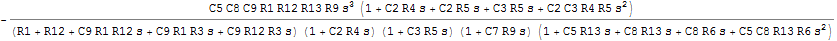I wasn't lucky enough to study under Middlebrook (not here anymore) but I'm familiar with his work. He would say that the overall gain expression is a high entropy expression.

It became obvious after a while that the TS didn't know how to use complex arithmetic. He also didn't need to know what each part does beyond seeing that there are high pass parts and low pass parts. Without using phasors the only thing he could do is to convert those resistor-capacitor pairs into impedance magnitudes and use those in place of complex impedances. He assumed a frequency of 1 Hz (which he was able to justify) then analyzed the various parts and multiplied the individual gains to get an estimate of the overall gain. His final result was within 6 db of the correct value. Not too bad for an estimate by someone who didn't know how to use phasors.The work you posted is well beyond the TS, as is the nodal analysis I posted. Maybe if he continues his studies he will someday be able to look back at this thread and say to himself "Now I see what they were trying to explain!".

•DaveE